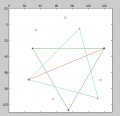Reflection of a Point Over a Line

MrChips

Joined Oct 2, 2009
19,160
Your diagram looks correct.
Your x-values appear to be correct.

Edit: My initial values were:

30, 30
120, 30
75, 107

25, 69
89, 5
112, 92

Here are my values for all six points:

55.9606 93.2372
120.0000 30.0000
33.8773 6.8293

25.0000 69.0000
115.5079 69.5705
70.7442 -8.4950MATLAB plots the 0,0 origin at left,bottom corner. The figure is flipped to match yours.

Here is the MATLAB code using matrix arithmetic:
Code:
% 2017.04.28 - MrChips
% define points of two triangles p and q
p1 = [ 30  30];
p2 = [120  30];
p3 = [ 75 107];

q1 = [ 25 69];
q2 = [ 89  5];
q3 = [112 92];

p = [p1;p2;p3];
q = [q1;q2;q3];

all = [p;q];  % all points

% shift
[nrows, ncols] = size(all);
Shift = ones(nrows,1) * q1;
all = -Shift + all;

% rotate
a = atan((p2(2)-q1(2))/(p2(1)-q1(1)));
Rotate = [[cos(a) -sin(a)]; [sin(a) cos(a)]];
all = all * Rotate;

% reflect about p2-q1 line
Reflect = ones(nrows,2);
Reflect(:,2) = -Reflect(:,2);
all = Reflect .* all;

% rotate back
a = -a;
Rotate = [[cos(a) -sin(a)]; [sin(a) cos(a)]];
all = all * Rotate;

% shift back
all = Shift + all

Last edited:

zulfi100

Joined Jun 7, 2012
495
Hi,
Thanks Mr. Chips. I also verified the values using the sited told by Mr. Tesla23. But i still want to solve using his method.

Zulfi.

MrChips

Joined Oct 2, 2009
19,160
The beauty with matrix arithmetic is you can do the calculation on any number of points at the same time.
If you wish to do the calculation for one point (px, py), try a straight forward geometry approach.

Step 1 : Find the slope m of the line (x1r, y1r) - (x2, y2)
Step 2 : Find the equation of the line (x1r, y1r) - (x2, y2)
Step 3: Find the equation of the line passing through (px, py) rotated pi/2
Step 4: Find the intersection (x0, y0) of the two lines
Step 5: Find the displacement between (px, py) - (x0, y0), i,e. (x0 - px), (y0 - py)
Step 6: Add this displacement to (x0, y0)

Tesla23

Joined May 10, 2009
374
Hi,
Thanks Mr. Chips. I also verified the values using the sited told by Mr. Tesla23. But i still want to solve using his method.

Zulfi.
I'd suggest you should do it using a method you understand. I gave you ideas as to how you can solve this using vectors without giving you the explicit answer, but I now suspect you haven't covered vectors yet.

I can give you an explicit formula for reflecting the point (x,y) in the line through (x1, y1) and (x2, y2) derived using vectors:

compute $\alpha=2\frac{(x - x_1)(x_2 - x_1) + (y - y_1)(y_2 - y_1)}{(x_2 - x_1)^2 + (y_2 - y_1)^2}$

then the reflected point is (xr, yr) where:

$x_r = (2-\alpha)x_1 + \alpha x_2 - x$
$y_r = (2-\alpha)y_1 + \alpha y_2 - y$

but you won't learn anything just by plugging in your numbers. At some stage you will probably learn about vectors, and you will hopefully realise that co-ordinate geometry would have been a lot easier if they had only taught them to you first.

No method is better than the other, and numerically if you collapsed and simplified the matrix equations you should end up with the same expressions. The more tools you have the better, I find vectors are simpler for this type of problem (the expressions above can be derived with one simple diagram and two lines of algebra), not so good for rotations - there I tend to use complex numbers if I can - simply because I find it easier to get the sign right. If I am implementing it in a computer where I have in-built support for rotation matrices - of course I use them.